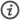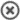# The function plotter...... is a simple tool to plot curves of functions (rsp. their derivatives or integrals) in cartesian coordinates. Use it to to display common mathematical functions or expressions as jpeg, png or gif image.

The lower tab allows to calculate tables of function values (in csv, text or laTeX format) and get a hyperlink to reproduce an image on the fly (using an URL or QRcode).

f(x) +C: color #
show: f(x)   df/dx   ∫f(x)dx
range: to         legend
g(x) +C: color #
show: g(x)   dg/dx   ∫g(x)dx
range: to         legend
h(x) +C: color #
show: h(x)   dh/dx   ∫h(x)dx
range: to         legend
hull function=
substitute Q=pointsDefine named points to be plotted into the curve:
add or remove points with +/-.
color #
plot properties
 width: height: border x-axis from to y-axis from to axis lines intervals x-axis: y-axis: caption grid lines: x-axis y-axis show grid dashes length: x-axis y-axis dashes decimal places: gap at origin: graph thickness: variable name: log. scale x: No 2 e 10 100 or log. scale y: No 2 e 10 100 or

colors
background #
caption # grid # gap #
image properties
image type:
°
apply filter

Enter an expression and some variable values (comma or space-separated) and press calculate to produce sample points.Enter some variable values and a function term to be calculated, the functions used in the plot above can be copied using one of the buttons labeled f(x), g(x) or h(x). Variables will usually be simple numbers, separate multiple input values (x) by commas or spaces.

Select one of the checkboxes to chose a format for output:

• results - a vector-like string of function values, separated by spaces
• table - a html table including variable values and results
• csv - colon separated values including variable values
• latex - code to layout a table including variable values and results in laTeXcalculate discrete values:
 Function: Input value(s):Load & save:

In case you want to link to the graph or cannot use one of the image formats provided, the graph in the plotter can be reproduced any time by the following URLEvery image drawn by plotter can be dynamically reproduced by a simple call to plotter's image creating part (called graph.php). All the information needed is placed in the query string appended to its URL. So, wehenever you call this URL (including the querystring), an image file of the plot above will be returned.

Because this is an ugly beast, I added my own URL shortener - the link via oeshort.de is equivalent to the long one.

Of course, this Information can be used to load an existing plot, e.g. to modify some of its settings - that is what the button labeled Load graph is for.full URL to your image file